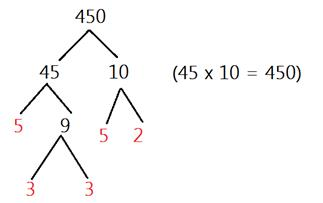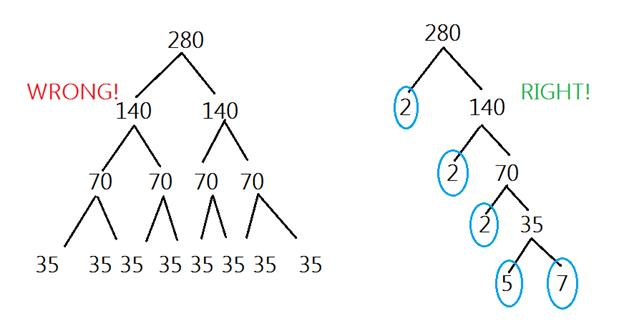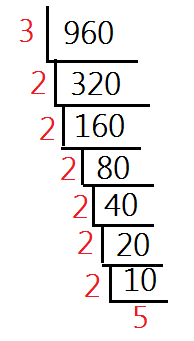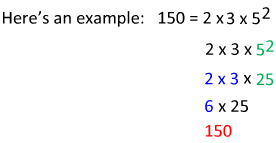# Prime Factorization

The prime factorization of a number is the product of prime factors that make up that number. Let's take a look at what that means.

Remember that product means the answer to a multiplication problem.

Factors are numbers that can be multiplied together to make another number. For example, 3 and 12 are a factor pair of 36.

Prime numbers are numbers that have exactly two factors, 1 and itself (i.e. 2, 3, 5, 7, 11,....).

So, prime factorization is writing the prime numbers that will multiply together to make a new number as a multiplication problem.

Here are some examples:

Ex. 1) 15 = 3 x 5

Ex. 2) 27 = 3 x 3 x 3 = 33 (We wouldn't want to write 3 x 9 because 9 isn't prime.)

Ex. 3) 48 = 2 x 2 x 2 x 2 x 3 = 24 x 3

It can be difficult to write the prime factorization of a large number. So there are a few methods you can use to do this.

Method 1:
The Factor Tree

This method is great because you can start with any factor, not just prime factors. Then you continue to branch out until you only have prime factors left. Here is an example:We can start with 45 x 10 because 45 x 10 = 450. Each number branches to a factor pair. At the end of the branches, we are left with the prime factors of 450.
So the prime factorization of 450 = 2 x 3 x 3 x 5 x 5
We can rewrite this using exponents.

450 = 2 x 32x 52

The most common mistake that is made when using the factor tree is that some people pick to numbers that add to make the value.So the prime factorization of 280 is 23 x 5 x 7.

Method 2: Upside Down Division

We can also place a number in the upside down division bar to get the prime factorization.

The number goes on the inside and the prime factors are written on the outside. We would divide the given number by the prime to get another new number to divide. This step would keep getting repeated until you are left with a prime. Here is an example.From the upside down division, we have all of the prime factors. So now, we can write the prime factorization. 960 = 26 x 3 x 5

If you wonder if your prime factorization is correct, you simply check it. Checking your answer is easy. All you do is multiply all the primes and see if you get back to the original number.Here, we ended where we started. Therefore, our prime factorization of 150 is correct.

Let's Review: Prime Factorization is the product of primes that could be multiplied together to make the original number. Two possible ways of getting the list of the primes include a factor tree and upside down division. You can check to see if your answer is right by solving your product of primes.